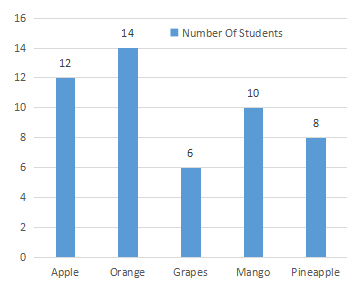Question 4

# The bar graph represents the favorite fruits of Grade 4 students.What is the total number of students in the class?Solution

The correct option is B.

The total number of students is 12+ 14+ 6 + 10 + 8= 50